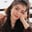Related Tags

math.rect
python programming
communitycreator

# What is cmath.rect(r, phi) in Python?Fouzia Bashir

The cmath.rect() method is used to convert polar coordinates to rectangular coordinates.

The cmath library in Python is used for complex number mathematics.

Polar to Rectangular Coordinate System Transformation

### Syntax

math.rect(r, phi)


### Parameters

• r: The modulus of a complex number in the complex plane.
• phi(ϕ): The phase of a complex number.

### Return value

Complex number: it will return a complex value, which will represent a rectangular form.

### Code

It will return a complex number in pair form (X+Yj).

• Example 1: When both are non zero, it will return a complex number.
• Example 2: When the phase is zero phi=0.
• Example 3: When the modulus is zero r=0.
# include the Library
import cmath
# r=4, phi=20
# Printing the result on console
print(cmath.rect(4,20))

RELATED TAGS

math.rect
python programming
communitycreator

CONTRIBUTORFouzia Bashir
RELATED COURSES

View all Courses

Keep Exploring

Learn in-demand tech skills in half the time